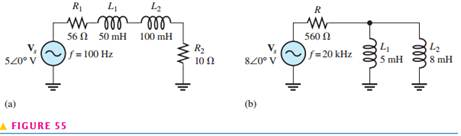Create an Account

Home / Questions / Determine the impedance magnitude and phase angle in each circuit in Figure 55 Draw the im...

Determine the impedance magnitude and phase angle in each circuit in Figure 55 Draw the impedance diagrams.

Determine the impedance magnitude and phase angle in each circuit in Figure 55. Draw the impedance diagrams.Jul 31 2020 View more View LessSubscribe To Get Solution